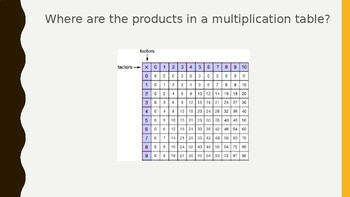# Topic 5- Fluently Multiply and Divide within 100Subject
Resource Type
File Type

Presentation (Powerpoint) File

(1 MB|37 pages)
Product Rating
Standards
• Product Description
• StandardsNEW

This is 7 days worth of whole class (or small group if you choose) questions that align with Topic 5 from Envisions math for 3rd grade. Each day has about 4 questions per day. Learning targets in "I can... so I can..." format are aligned to each day. I use these for whole group and then break into small groups. You can align students independent work to each days lesson using the Envision student edition. PowerPoint is editable as well!

Fluently multiply and divide within 100, using strategies such as the relationship between multiplication and division (e.g., knowing that 8 × 5 = 40, one knows 40 ÷ 5 = 8) or properties of operations. By the end of Grade 3, know from memory all products of two one-digit numbers.
Understand division as an unknown-factor problem. For example, find 32 ÷ 8 by finding the number that makes 32 when multiplied by 8.
Apply properties of operations as strategies to multiply and divide. Examples: If 6 × 4 = 24 is known, then 4 × 6 = 24 is also known. (Commutative property of multiplication.) 3 × 5 × 2 can be found by 3 × 5 = 15, then 15 × 2 = 30, or by 5 × 2 = 10, then 3 × 10 = 30. (Associative property of multiplication.) Knowing that 8 × 5 = 40 and 8 × 2 = 16, one can find 8 × 7 as 8 × (5 + 2) = (8 × 5) + (8 × 2) = 40 + 16 = 56. (Distributive property.)
Determine the unknown whole number in a multiplication or division equation relating three whole numbers. For example, determine the unknown number that makes the equation true in each of the equations 8 × ? = 48, 5 = __ ÷ 3, 6 × 6 = ?.
Total Pages
37 pages
N/A
Teaching Duration
30 minutes
Report this Resource to TpT
Reported resources will be reviewed by our team. Report this resource to let us know if this resource violates TpT’s content guidelines.